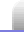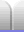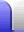#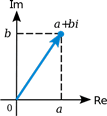### Real and Imaginary

Complex numbers are an extension to real numbers in a similar way to how negative numbers are an extension to positive numbers. Complex numbers allow us to do things that the smaller set of numbers doesn't let us do, such as finding the square root of a negative number. It means we can find solutions to quadratic equations where the discriminant is negative.

Complex numbers have two components, a real part and an imaginary part. If z is a complex number, z = a + bi, where a and b are real numbers and i satisfies i2 = -1.

Note that the equation i2 = -1 has two solutions, which we call i and -i. We have no way of telling which is which, but it doesn't matter as long as we're consistent in which we use. Everything still works if we consistently use the other.

### Argand Diagram & Polar Coordinates

The real and imaginary components of a complex number are completely independent, which means complex numbers can be drawn as points on two axes at right angles to each other. This is called an Argand diagram, which looks like a Cartesian plane but with different axes. On an Argand diagram the horizontal axis is real numbers, the vertical axis is imaginary numbers. Every complex number can be represented as a point somewhere on the Argand diagram.

Complex numbers are often described using polar notation, which is two numbers that describe where the point is:

• The modulus (or magnitude), r, is the distance to the point from the origin.
• The argument, θ, is the angle anticlockwise from the postive real axis. -180° < θ ≤ 180°.

This can be written using Euler's formula re = r(cos θ + i sin θ). This is often abbreviated as r cis θ. The real and imaginary components are directly related to the parts of this formula.

a = r cos θ
b = r sin θ

For example:

• The complex number 1 + i, which is the point (1,1), converts to √2 eiπ/4.
• The complex number 3 + 4i, which is the point (3,4), converts to 5e0.927i.
• The complex number -2 - 2i, which is the point (-2,-2), converts to √8cis-¾π.

Polar notation makes the maths for things like flight navigation systems, and AC impedance and phase shifts much easier. It also makes raising complex numbers to high powers particularly easy. Using complex numbers is really really useful in certain situations.

### Example Problems

#### 1. Draw the graph of | z + 2i | ≤ 3.

In the same way that adding a number to x or y shifts a graph in a direction on that axis opposite to the number added, adding a number to z also shifts the graph in the opposite direction.

Compare:

y = x2
y = (x – 1)2
y – 1 = x2

• The first equation is a parabola with its lowest point at the origin – the green graph.
• In the second equation, subtracting a number from x (before squaring or whatever) shifts the parabola to the right – the blue graph.
• In the third equation, subtracting a number from y shifts the graph up – the red graph. It might make more sense (and be more familiar) writing it as y = x2 + 1.So let's take a look at the complex equation for this question.

| z | ≤ 3
| z + 2i | ≤ 3

The graph of | z | ≤ 3 is a disc (a solid circle) centred on the origin – the blue disc in the graph below. It's the set of points which have a magnitude less than or equal to 3, or to put it a different way, the set of points within or equal to a radius of 3 from the origin.

Adding 2i before taking the magnitude of the left side of the equation shifts the disc down 2 – the red disc in the graph.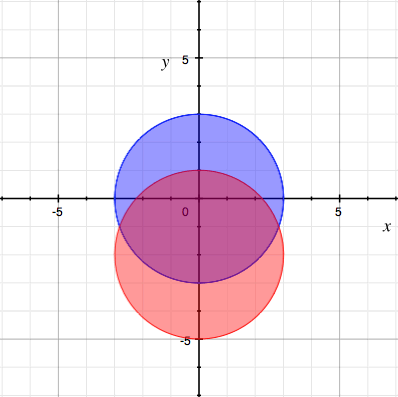#### 2. Find the greatest and least values of | z – 3 – 2i | given | z + 2i | ≤ 3.

This is asking for the greatest and least values of | z – 3 – 2i | that fall within the disc from the previous example. These will be two real numbers. This is not asking for the value of z, a complex number.

| z – 3 – 2i | is always positive, so without the restriction of | z + 2i | ≤ 3 its least value would be zero, when z = 3 + 2i. But | 3 + 4i | = 5, so 3 + 2i lies outside the restriction circle.

The least value of | z – 3 – 2i | will be the nearest point on (or within) the circle to the point 3 + 2i. I can find that point if I take a line from 3 + 2i through the centre of the circle at -2i. The reason it's the nearest point to the centre of the circle is the line and the circle will be perpendicular at their intersection because the line is a radius of the circle, and either side of the intersection the circle is further away from 3 + 2i than the intersection is.

The point 3 + 2i makes a 3, 4, 5 triangle from the centre of the circle. That's convenient. The point of intersection forms a similar triangle from the centre of the circle and has a hypotenuse length of 3 (the radius of the circle), so taking 3/5 = 0.6 of the other two sides gives a 1.8, 2.4, 3 triangle and thus gives the location of the point of intersection, 1.8 + 0.4i.

Therefore the least value of | z – 3 – 2i | that fits within the restriction is:

 =  = | 1.8 + 0.4i – 3 – 2i | | -1.2 – 1.6i | 2

(The complex number -1.2 – 1.6i happens to be a scale multiple of a 3, 4, 5 triangle. See if you can figure out why.)

The further along the line we go the greater the value of | z – 3 – 2i |. This means its greatest value is a continuation of that same line through the centre of the circle to the other side, giving the point of intersection at the bottom left of the circle, -1.8 – 4.4i, the point on the circle furthest from 3 + 2i.

Therefore the greatest value of | z - 3 - 2i | that fits within the restriction is:

 =  = | -1.8 – 4.4i – 3 – 2i | | -4.8 – 6.4i | 8

(The complex number -4.8 – 6.4i is again a scale multiple of a 3, 4, 5 triangle.)

Graphically, the solutions are the intersections of the circle and a straight line through its centre from 3 + 2i. Click for a larger version.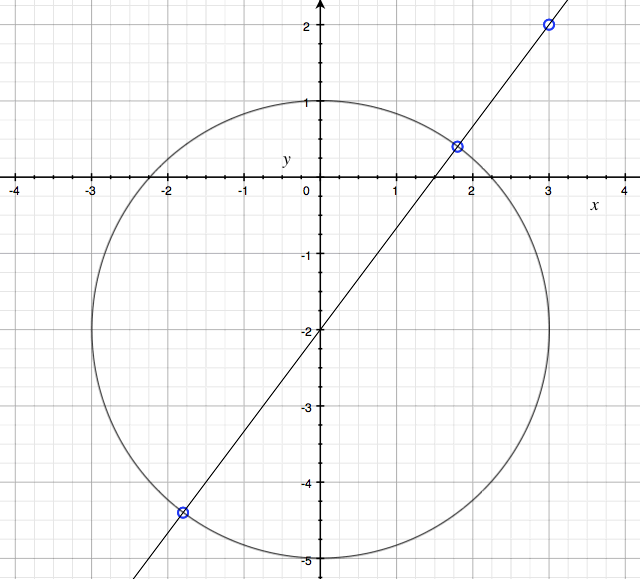Instead of a line, it would be more accurate to represent | z – 3 – 2i | with two circles centred at 3 + 2i, one representing the least value of z which just touches the other circle at its top right boundry, the other circle representing the greatest value of z which just touches the other circle at its bottom left boundry.

### Complex Weirdness

Let's start with something simple, change a sign, then throw in an imaginary number, and see what we get.

11 = 1 (All good so far.)

1-1 = 1 (That negative sign in the exponent just means 1 over the number. 1/1 = 1, so it gives the same result.)

1i = 1 (Raising 1 to an imaginary exponent hasn't changed its value.)

1-i = 1 (It's still 1/1 = 1, but also remember that we can consistently change i for -i and still get the same result.)

(-1)-i = 23.14069... (Er, what?)

To understand this last result, we need to know Euler's identity, a special case of Euler's formula (mentioned above).

e = -1

Euler's identity has been called the "most beautiful theorem in mathematics". You can accept it on faith, or you can try to understand it, first making sure you understand Argand diagrams (mentioned above), then by watching this video. Either way, let's substitute Euler's identity into the previous equation.

(-1)-i = (e)-i = e-i²π = e-(-1)π = eπ = 23.14069...

OK, that makes much more sense. FWIW eπ is Gelfond's constant.Home Astronomy Chemistry Electronics & Computers Mathematics Physics Field Trips Turn on javascript for email link